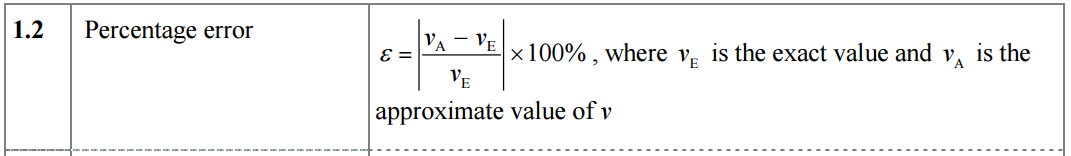### 02. Number

IBO Syllabus
Topic 1 - Number and Algebra
1.1 : Natural numbers; integers; rational numbers; and real numbers.
1.2: Approximation: decimal places, significant figures. Percentage errors. Estimation.
1.3: Expressing numbers in the standard form. Operations with numbers in this form.
1.4: SI (Système International) and other basic units of measurement: for example, kilogram (kg), metre (m), second (s), litre (l), metre per second (m s –1 ), Celsius scale.

Textbook Reference
1.1 The number sets p3-11
- Natural numbers
- The set of Integers
- The set of rational numbers
- The set of real numbers
1.2 Approximations and error p11-21
- Rounding numbers to the nearest unit, 10, 100, 1000 etc
- Rounding numbers to a given number of decimal places (dp)
- Rounding numbers to a given number of significant figures (sf)
- Estimation
- Percentage errors
1.3 Standard Form p22-25
- Calculations with numbers expressed in standard form
1.4 SI units of measurement p25-34
- SI units of measurement
- SI prefixes
- Area and Volume SI units
- Non-SI units accepts in the SI
- Temperature
Review exercises p34-37: All questions

IBO Formula Booklet